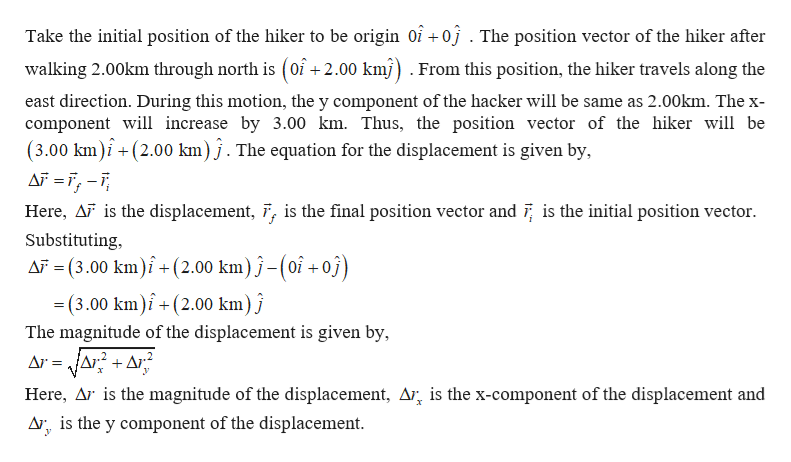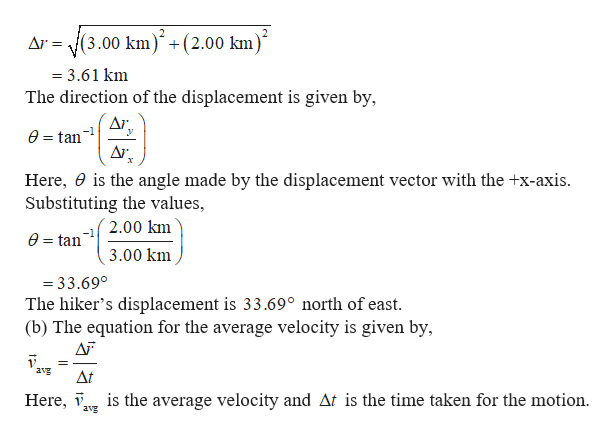# A hiker walks 2.00 km north and then 3.00 km east, all in2.50 hours. Calculate the magnitude and direction of thehiker’s (a) displacement (in km) and (b) average velocity (inkm/h) during those 2.50 hours. (c) What was her averagespeed during the same time interval?

Question
30 views

A hiker walks 2.00 km north and then 3.00 km east, all in
2.50 hours. Calculate the magnitude and direction of the
hiker’s (a) displacement (in km) and (b) average velocity (in
km/h) during those 2.50 hours. (c) What was her average
speed during the same time interval?

check_circle

Step 1

Take the north direction to be +y direction and east direction to be +x direction.help_outlineImage TranscriptioncloseTake the initial position of the hiker to be origin oi +0j . The position vector of the hiker after walking 2.00km through north is (oî +2.00 kmj) . From this position, the hiker travels along the east direction. During this motion, the y component of the hacker will be same as 2.00km. The x- component will increase by 3.00 km. Thus, the position vector of the hiker will be (3.00 km)i + (2.00 km) j . The equation for the displacement is given by, AF =ï; -T, Here, AF is the displacement, 7, is the final position vector and 7; is the initial position vector. Substituting, AF = (3.00 km)î + (2.00 km) ĵ–(oî + oj) = (3.00 km)î + (2.00 km) ĵ %3D The magnitude of the displacement is given by, Ar = JAr? + Ar? Here, Ar is the magnitude of the displacement, Ar, is the x-component of the displacement and Ar, is the y component of the displacement. fullscreen
Step 2

Substituting the...help_outlineImage TranscriptioncloseAr = J(3.00 km)´ +(2.00 km = 3.61 km The direction of the displacement is given by, Δι. У e = tan Ar. Here, 0 is the angle made by the displacement vector with the +x-axis. Substituting the values, 2.00 km -1 e = tan 3.00 km = 33.69° The hiker's displacement is 33.69° north of east. (b) The equation for the average velocity is given by, AF %3D avg At Here, i is the average velocity and At is the time taken for the motion. avg fullscreen

### Want to see the full answer?

See Solution

#### Want to see this answer and more?

Solutions are written by subject experts who are available 24/7. Questions are typically answered within 1 hour.*

See Solution
*Response times may vary by subject and question.
Tagged in

### Physics